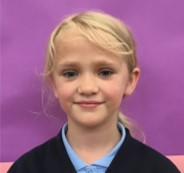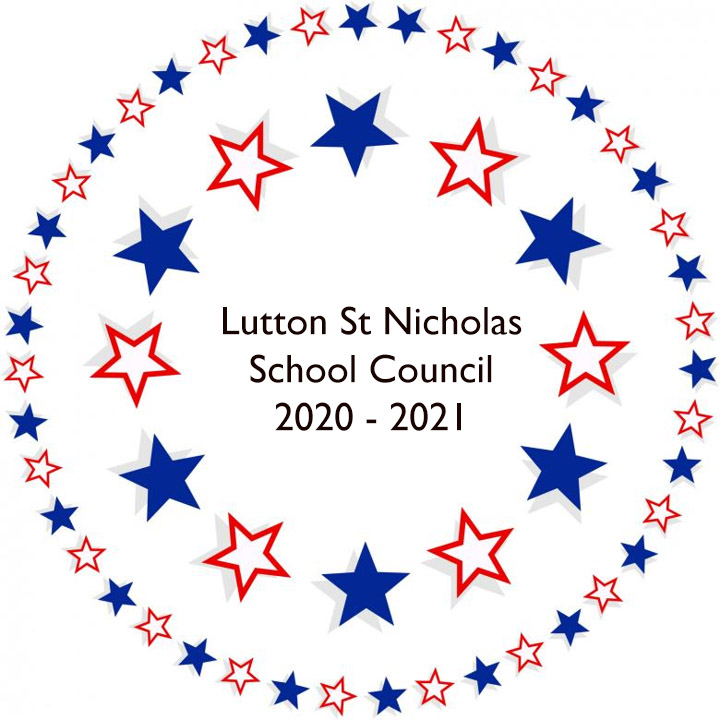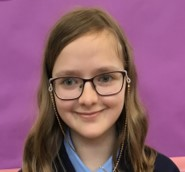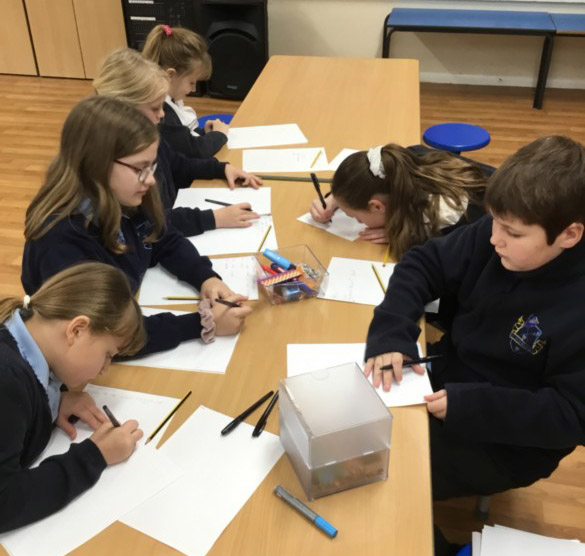Marriots Gate, Lutton, Spalding, Lincolnshire PE12 9HN Tel: 01406 363 392 Email enquiries@lutton.lincs.sch.uk

#School CouncilWe are the student voice

We represent the school

We listen to the students and help make changesWe create fundraising ideas for the school

We have regular meetings with our Education Care Officer

We have ideas to help make our school a better place

## Student QuestionnaireOne of the first tasks given to the school council was to get the children’s voice. Unfortunately due to corona virus, we currently are operating with 2 bubbles, meaning ks1 and ks2 cannot mix. The school councillors decided they would create a questionnaire for each student to complete instead. The questionnaire results are in and have been compiled below. You can also download the Questionnaire.

 Class Question 1 – Do you like this school? Question 2 – Are you comfortable with the school rules? Question 3 – Do you like the school dinners? Question 4 – Do you have any friends at school? Question 5 – Do you feel you can talk to your class teacher? Question 6 – Do you find the work ok that your teacher sets you? Scale to rate the school. 1 – poor to 10 – excellent. Caterpillars Y = 12 N = 0 Y = 9 N = 3 Y = 9 N = 1 N/A = 2 Y = 12 N = 0 Y = 12 N = 0 Y = 12 N = 0 10 = 10 8 = I 6 = 1 Penguins Y = 28 N = 0 Y = 25 N = 3 Y = 16 N = 0 N/A = 12 Y = 28 N = 0 Y = 28 N = 0 Y = 26 N = 2 10 = 17 9 = 6 8 = 3 5 = 1 1 = 1 Elephants Y = 32 N = 1 Y = 30 N = 1 Don’t know = 2 Y = 23 N = 8 N/A = 2 Y = 31 N = 2 Y = 28 N = 5 Y = 26 N = 7 10 = 19 9 = 4 8 = 3 7 = 3 6 = 1 5 = 2 1 child did not score. Dolphins Y = 23 N = 0 Y = 22 N = 0 Don’t Know = 1 Y = 10 N = 4 N/A = 9 Y = 23 N = 0 Y = 17  N = 6 Y = 21 N = 1 10 = 2 9 = 4 8 = 9 7 = 2 6 = 4 4 = 1 1 child did not score.